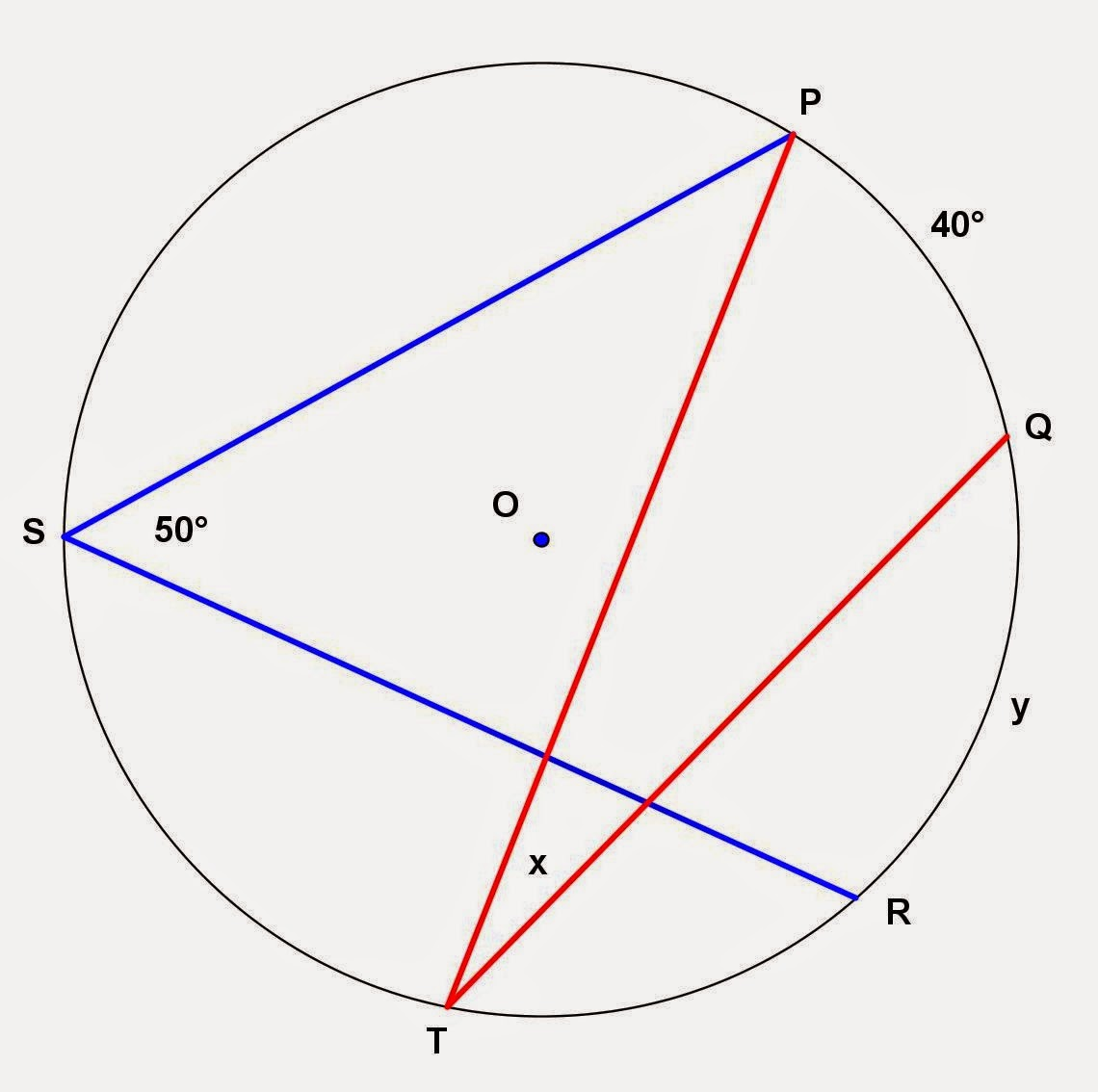## Monday, December 8, 2014

### Circle and Inscribed Angle Problems

Category: Plane Geometry

"Published in Newark, California, USA"

Find the values of x and y in the given figurePhoto by Math Principles in Everyday Life

Solution:

The given figure consists of two inscribed angles. Inscribed angle is an angle of which the vertex is located along the arc of a circle. The sides of the inscribed angles are the chords of a circle. ∠PSR and ∠PTQ are inscribed angles of circle O.

Let's consider ∠PTQ and draw the line segments OP and OQ as followsPhoto by Math Principles in Everyday Life

If a theorem says "The measure of an inscribed angle is equal to half the measure of its intercepted arc.", then the value of x which is the measure of ∠PTQ is

Let's consider ∠PSR and draw the line segments OP and OR as followsPhoto by Math Principles in Everyday Life

If a theorem says "The measure of an inscribed angle is equal to half the measure of its intercepted arc.", then the value of y which is the measure of arc QR is